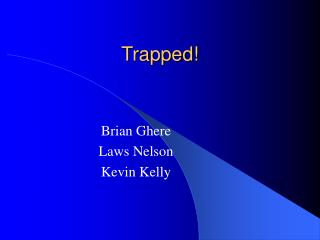DownloadDownload PresentationTrapped!

# Trapped!

Télécharger la présentation## Trapped!

- - - - - - - - - - - - - - - - - - - - - - - - - - - E N D - - - - - - - - - - - - - - - - - - - - - - - - - - -
##### Presentation Transcript

1. Trapped! Brian Ghere Laws Nelson Kevin Kelly

2. Overview • Ball rolls down a ramp • Ball falls out and hits another ramp changing its direction • Ball rolls down the other ramp and sets off a mouse trap • PVC pipe • Mouse trap • Wood base

3. Energy Conversions • 1st Conversion: Ball rolls down the first ramp changing energy from potential to kinetic. • Mgh=(1/2)mv^2+mgh • (5g)(9.81m/s^2)(.2699m)=(.5)(5g)v^2+(5g)(9.81m/s^2)(.2223m) • V=.966m/s • 2nd Conversion: Ball hits the second ramp causing energy loss. • (1/2)mv^2=mgh+Eloss • (.5)(5g)(.966m/s)^2=(5g)(9.81m/s^2)(.0254m)+Eloss • Eloss=.109J • 3rd Conversion: Ball rolls down the second ramp. • Mgh=(1/2)mv^2+mgh • (5g)(9.81m/s^2)(.2223m)=(.5)(5g)v^2+(5g)(9.81m/s^2)(.0191m) • V=2.00m/s

4. Conclusion • 95% success rate • Developed good team working skills • Real world application of conservation of energy equations